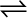7.15 At 700 K, equilibrium constant for the reaction:

H2 (g) + I2 (g)2HI (g)

is 54.8. If 0.5 mol L–1 of HI(g) is present at equilibrium at 700 K, what are the concentration of H2(g) and I2(g) assuming that we initially started with HI(g) and allowed it to reach equilibrium at 700?

It is given that equilibrium constant ${K}_{c}$ for the reaction
Therefore, at equilibrium, the equilibrium constant for the reaction
$K{\text{'}}_{c}$ for the reaction

Let the concentrations of hydrogen and iodine at equilibrium be
Hence, at equilibrium,